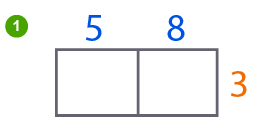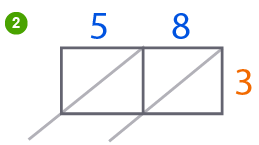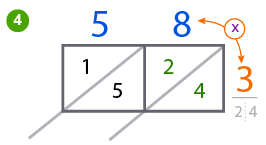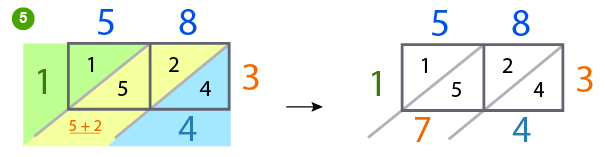# Lattice multiplication worksheets PDF - Printable multiplication tests

## Lattice method multiplication examples and test

To learn how to do or revising multiple digits with multiplication is fairly simple with these lattice multiplication worksheets PDF.
Practice on our Printable multiplication tests consists of lattice multiplication worksheets 3 by 3, lattice multiplication worksheets 3 by 2, lattice multiplication worksheets 4 by 2 and more printable grids and templates.

## Multiplication Methods Activities

### IMPORTANT FACTS ABOUT LATTICE MULTIPLICATION

Feel excited when multiplying large numbers by engaging in our lattice multiplication worksheets PDF. You may not always walk along with a calculator to help solve 2 and more digit number.

In light of this, the lattice method is a very simple tip to enhance easy multi-digit multiplication. So to speak, we offer printable multiplication tests that with the use of the lattice method, it'll empower a constant practice of multiplying large numbers.

Lattice method multiplication examples and test will showcase a very simple and effective way to solve multi-digit numbers.

In actual fact, our Printable multiplication tests consists of lattice multiplication worksheets PDF → 2 by 1; → 2 by 2; → 3 by 3; → 4 by 3 and more printable grids and templates.

Using the lattice method to multiply large numbers, requires just a very little technique. This technique usually begins with the construction of a lattice (grid), sized to fit the multiplicand and the multiplier.

### Example 1.   2 digit multiplication: lattice method | Multiplying 2 digit by 1 digit

Multiply 58 x 3

Firstly, we draw a grid of 2 columns (for 58) and 1 row (for 3), then place 5 and 8 at the top of the grid then 3 on the right hand side of the grid.Secondly, draw a diagonal path along each cell down until it goes out of the cell.Next, we multiply the first number of the multiplicand, and the first of the multiplier. Since we only have one multiplier, we'll multiply just once.

• when we multiply 5 x 3 = 15, we place 1 on the upper triangle of the first cell and 5 on the lower triangle of its cell.• in the same light, 8 x 3 = 34. We place 2 on the upper triangle of the second cell, and 4 on the lower triangle.Furthermore, we start adding the numbers on the same diagonal path, from the last triangle of the last cell.
→ 4 + nothing = 4
→ 2 + 5 → = 7
→ 1 + nothing = 1Very good. Now finally, we combine the digits of the answer from top of the lattice to bottom.

58 x 3 = 174

### Example 2.   3 digit multiplication: lattice method | Multiplying 3 digit by 2 digit

Multiply 119 x 23

Yeah. Simple.

We gonna follow the steps below.

Firstly, as usual, we draw our grid of 3 columns (for 119) and 2 rows (for 23), then place 119 at the top of each cell and 23 on the right hand side of the grid.

Secondly, we draw diagonal paths along each cell down until it goes out of the cell.Thirdly, we begin multiplying.

2 →     (1)   2 x 1 = 2     (2)   2 x 1 = 2     (3)   2 x 9 = 18

We now go to our second multiplier

3 →     (1)   3 x 1 = 3     (2)   3 x 1 = 2     (3)   3 x 9 = 27

We should note that when a product is less than 10, we place a zero (0) on the upper triangle, then the product on the lower triangle of its cell, but when it is more than 10, we write the first digit of the answer on the upper triangle of the cell, and the second digit on the lower triangle.Fourthly, we commence addition. We add numbers sloping down on the diagonal path, hence

• 7 + nothing = 7

• 3 + 2 + 8 = 13,

we write 3 and carry 1 to the next path.

• 3 + 0 + 2 + 1 + (1) = 7

• 0 + 2 + 0 = 2

• 0 + nothing = 0Wow! Perfect. Our answer is 02737

So, 119 x 23 = 2737

•